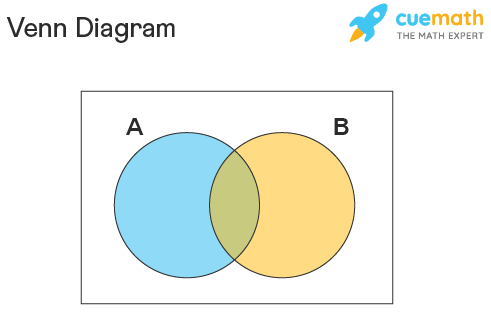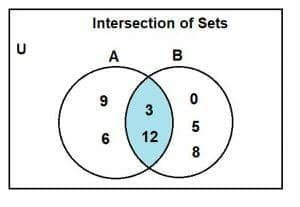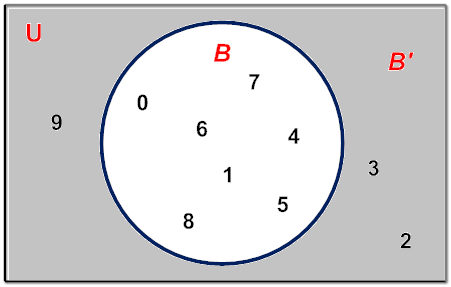# Sets and Operations of Set

Introduction

### SET

- in mathematics, are an organized collection of objects and can be represented in set-builder form or roster form. Usually, sets are represented in curly braces {}, for example, A = {1,2,3,4} is a set. Also, check the set symbols here.

In sets theory, you will learn about sets and it’s properties. It was developed to describe the collection of objects. You will learn about the classification of sets here. The set theory defines the different types of sets, symbols and operations performed.

## Why are Sets Important?

Sets are the fundamental property of mathematics. Now as a word of warning, sets, by themselves, seem pretty pointless. But it's only when we apply sets in different situations do they become the powerful building block of mathematics that they are.

Math can get amazingly complicated quite fast. Graph Theory, Abstract Algebra, Real Analysis, Complex Analysis, Linear                           Algebra, Number Theory, and the list goes on. But there is one thing that all of these share in common: Sets.

At the end of the lesson students can:

a) identify the set and operations of set.

b) value the importance of the set and the use of its operations.

c) perform the different operation of sets and apply it in real life situation.

Process

Set operations is a concept similar to fundamental operations on numbers. Sets in math deal with a finite collection of objects, be it numbers, alphabets, or any real-world objects. Sometimes a necessity arises wherein we need to establish the relationship between two or more sets. There comes the concept of set operations.

There are four main set operations which include set union, set intersection, set complement, and set difference. This time, we will learn the various set operations, notations of representing sets, how to operate on sets, and their usage in real life.

## What are Set Operations?

A set is defined as a collection of objects. Each object inside a set is called an 'Element'. A set can be represented in three forms. They are statement form, roster form, and set builder form. Set operations are the operations that are applied on two more sets to develop a relationship between them. There are four main kinds of set operations which are:

• Union of sets
• Intersection of sets
• Complement of a set
• Difference between sets/Relative Complement

Before we move on to discuss the various set operations, let us recall the concept of Venn diagrams as it is important in understanding the operations on sets. A Venn diagram is a logical diagram that shows the possible relationship between different finite sets. It looks as shown below.## Basic Set Operations

Now that we know the concept of a set and Venn diagram, let us discuss each set operation one by one in detail. The various set operations are:

### Union of Sets

For two given sets A and B, A∪B (read as A union B) is the set of distinct elements that belong to set A and B or both. The number of elements in A ∪ B is given by n(A∪B) = n(A) + n(B) − n(A∩B), where n(X) is the number of elements in set X. To understand this set operation of the union of sets better, let us consider an example: If A = {1, 2, 3, 4} and B = {4, 5, 6, 7}, then the union of A and B is given by A ∪ B = {1, 2, 3, 4, 5, 6, 7}.

Example:
Given U = {1, 2, 3, 4, 5, 6, 7, 8, 10}
X = {1, 2, 6, 7} and Y = {1, 3, 4, 5, 8}
Find X ∪ Y and draw a Venn diagram to illustrate X ∪ Y.

Solution:
X ∪ Y = {1, 2, 3, 4, 5, 6, 7, 8} ← 1 is written only once.### Intersection of Sets

For two given sets A and B, A∩B (read as A intersection B) is the set of common elements that belong to set A and B. The number of elements in A∩B is given by n(A∩B) = n(A)+n(B)−n(A∪B), where n(X) is the number of elements in set X. To understand this set operation of the intersection of sets better, let us consider an example: If A = {1, 2, 3, 4} and B = {3, 4, 5, 7}, then the intersection of A and B is given by A ∩ B = {3, 4}.

Example

Consider a set A = {3, 6, 9, 12} and set B = {0, 3, 5, 8, 12}. Show the intersection between A and B through Venn diagram. Let set U = {set of whole numbers till 20}.

Solution:

The intersection between the two sets A and B can be determined as:

A = {3, 6, 9, 12}

And,

B = {0, 3, 5, 8, 12}

The intersection is:

A ∩ B = {3, 12}

The Venn diagram representation for the intersection between sets A and B is:### Set Difference

The set operation difference between sets implies subtracting the elements from a set which is similar to the concept of the difference between numbers. The difference between sets A and B denoted as A − B lists all the elements that are in set A but not in set B. To understand this set operation of set difference better, let us consider an example: If A = {1, 2, 3, 4} and B = {3, 4, 5, 7}, then the difference between sets A and B is given by A - B = {1, 2}.

Example:

1. If A = {2, 3, 4, 5, 6, 7} and B = {3, 5, 7, 9, 11, 13}, then find (i) A – B and (ii) B – A.

Solution:

According to the given statement; A = {2, 3, 4, 5, 6, 7} and B = {3, 5, 7, 9, 11, 13}

(i) A – B= {2, 4, 6}

(ii) B – A= {9, 11, 13}

### Complement of Sets

The complement of a set A denoted as A′ or Ac (read as A complement) is defined as the set of all the elements in the given universal set(U) that are not present in set A. To understand this set operation of complement of sets better, let us consider an example: If U = {1, 2, 3, 4, 5, 6, 7, 8, 9} and A = {1, 2, 3, 4}, then the complement of set A is given by A' = {5, 6, 7, 8, 9}.

Example:

Given= {0,1,2,3,4,5,6,7,8,9} and  B = {0, 1, 4, 5, 6, 7, 8}, find the complement of B.Answer: B= {2, 3, 9}, Thus B is the set of all numbers inthat are not in B.

## Examples of Set Operations

1. Example 1: In a school, every student plays either football or soccer. It was found that 200 students played football, 150 students played soccer and 100 students played both. Find how many students were there in the school using the set operation formula.

Solution: Let us represent the number of students who played football as n(F) and the number of students who played soccer as n(S). We have n(F) = 200, n(S) = 150 and n(F ∩ S) = 100. We know that,

n(F∪S) = n(F) + n(S) − n(F∩S)

Therefore, n(F∪S)=(200+150)−100

n(F∪S) = 350 − 100 = 250

Answer: The total number of students in school is 250.

2. Example 2: If A = {a, b, c, d, e}, B = {a, e, i, o, u}, U = {a, b, c, d, e, f, g, h, i, j, k, l, o, u}. Find

a) A ∪ B
b) A ∩ B
c) A′
d) A - B

Solution:

a) A ∪ B = {a, b, c, d, e, i, o, u}

b) A ∩ B = {a, e}

c) A' = {f, g, h, i, j, k, l, o, u}

d) A - B = {b, c, d}

## Practice Problems

Problem 1 :

In a survey of university students, 64 had taken mathematics course, 94 had taken chemistry course, 58 had taken physics course, 28 had taken mathematics and physics, 26 had taken mathematics and chemistry, 22 had taken chemistry and physics course, and 14 had taken all the three courses. Find how many had taken one course only.

Solution :

Step 1 :

Let M, C, P represent sets of students who had taken mathematics, chemistry and physics respectively

Step 2 :

From the given information, we have

n(M)  =  64, n(C)  =  94,  n(P)  =  58,

n(MnP)  =  28, n(MnC)  =  26, n(CnP)  =  22

n(MnCnP)  =  14

Step 3 :

From the basic stuff, we have

No. of students who had taken only Math

=  n(M) - [n(MnP) + n(MnC) - n(MnCnP)]

=  64 - [28 + 26 - 14]

=  64 - 40

=  24

Step 4 :

No. of students who had taken only Chemistry :

= n(C) - [n(MnC) + n(CnP) - n(MnCnP)]

= 94 - [26+22-14]

= 94 - 34

= 60

Step 5 :

No. of students who had taken only Physics :

=  n(P) - [n(MnP) + n(CnP) - n(MnCnP)]

=  58 - [28 + 22 - 14]

=  58 - 36

=  22

Step 6 :

Total no. of students who had taken only one course :

=  24 + 60 + 22

=  106

Hence, the total number of students who had taken only one course is 106.

Alternative Method (Using venn diagram) :

Step 1 :

Venn diagram related to the information given in the question:Step 2 :

From the venn diagram above, we have

No. of students who had taken only math  =  24

No. of students who had taken only chemistry  =  60

No. of students who had taken only physics  =  22

Step 3 :

Total no. of students who had taken only one course :

=  24 + 60 + 22

=  106

Hence, the total number of students who had taken only one course is 106.

Problem 2 :

In a group of students, 65 play foot ball, 45 play hockey, 42 play cricket, 20 play foot ball and hockey, 25 play foot ball and cricket, 15 play hockey and cricket and 8 play all the three games. Find the total number of students in the group (Assume that each student in the group plays at least one game).

Solution :

Step 1 :

Let F, H and C represent the set of students who play foot ball, hockey and cricket respectively.

Step 2 :

From the given information, we have

n(F)  =  65 , n(H)  =  45, n(C)  =  42,

n(FnH)  =  20, n(FnC)  =  25, n(HnC)  =  15

n(FnHnC)  =  8

Step 3 :

From the basic stuff, we have

Total number of students in the group is n(FuHuC).

n(FuHuC) is equal to

=  n(F) + n(H) + n(C) - n(FnH) - n(FnC) - n(HnC) + n(FnHnC)

Then, we have

n(FuHuC)  =  65 + 45 + 42 -20 - 25 - 15 + 8

n(FuHuC)  =  100

Hence, the total number of students in the group is 100.

Alternative Method (Using venn diagram) :

Step 1 :

Venn diagram related to the information given in the question :Step 2 :

Total number of students in the group :

=  28 + 12 + 18 + 7 + 10 + 17 + 8

=  100

So, the total number of students in the group is 100.

For further understanding you can read some of the related topic about set and its operation:

Evaluation

1. Find the union of each of the following pairs of sets.

(a) A = {2, 4, 6}

B = {1, 2, 3}

(b) P = {a, e, i, o, u}

Q = {a, b, c, d}

2. Find the intersection of each of the following pairs of sets.

(a) A = {1, 4, 9, 16}

B = {3, 6, 9, 12}

(b) C = {p, q, r, s}

D = {a, b}

3. If set A = {3, 4, 5, 6} and set B = {2, 4, 6, 8};

Find:

(a) A -B

(b) B - A

4. If U = {a, b, c, d, e, f} find the complement of the following:

(a) A = { }

(b) B = {c, d, f}

(c) D = {a, b, c, d, e, f}

(d) C = {a, b, d}

II. Using Venn Diagram show your answer to the given operation of sets.

If A= {0,5,7,3,8,1,3,} , B= {1,5,6,3,7,9} and C={ 4,2,9,5} then,

1. What is A U B ?

2. A U B U C ?

3. A ∩ B ∩ C

4. A-C

5. C'

III. It said that set is about an organized collection of objects. So in this part you have to gather information on your classmates about the gadgets/ technology they use to attend in our online class. Use Venn Diagram for the presentation of your answer.

Credits

References: